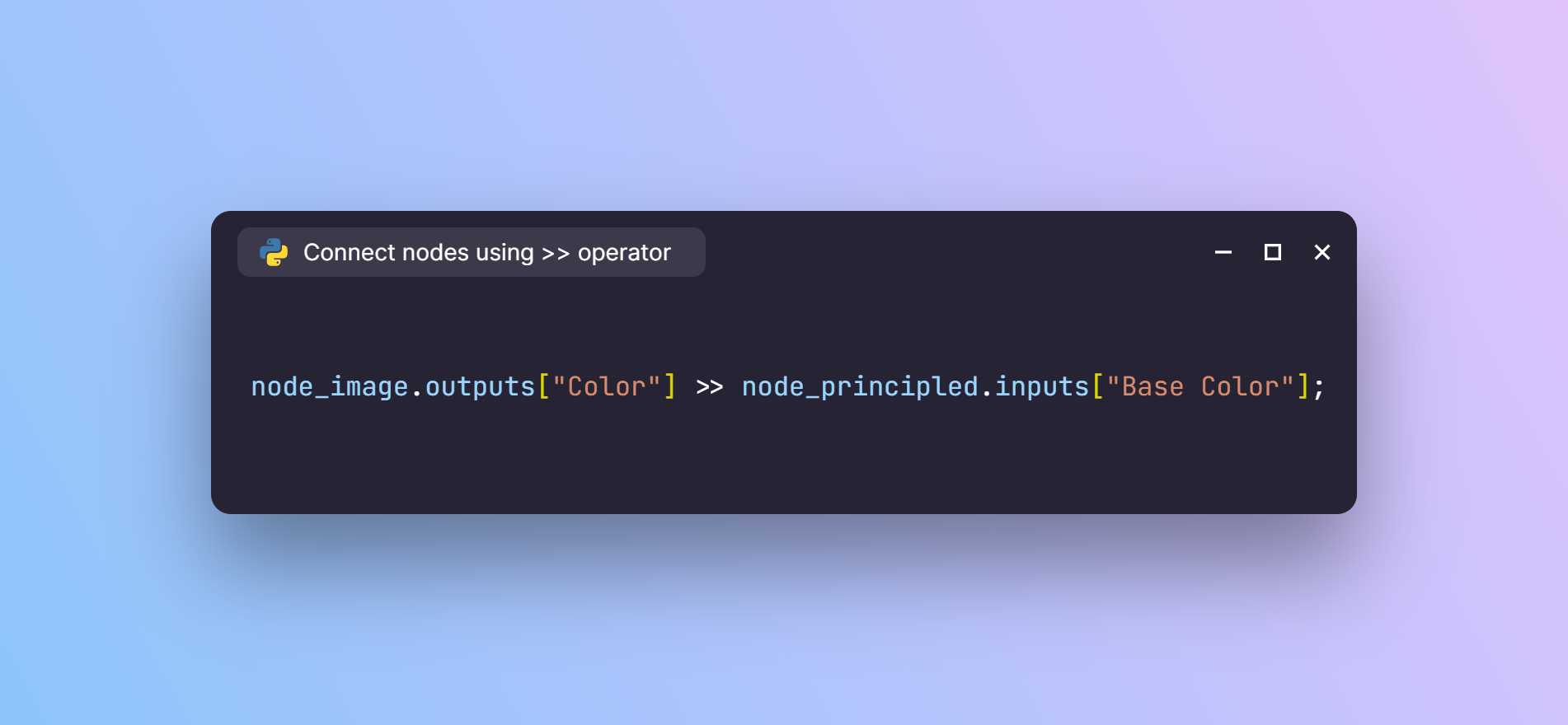I wrote a blog post, back in December, on building meaningful abstractions for the Blender API using arithmetic operators and context managers. I continued exploring some more ideas in that route. And this time, I tried something with the Blender’s node system.## Thoughts on the Blender API

If you are a Blender user who has never used the API, the first time you get acquainted with it, you’ll realize that the API has a lot of layers to it. And by layers I mean nested hierarchies that you’ll have to sift through to do the same things you do in the UI.

For example, if you want to connect two node sockets using the API, first you would do something like:

``````obj = bpy.data.objects['Cube']

node_tree = obj.data.materials['Material'].node_tree

node_image_tex = node_tree.nodes['Image Texture']
node_principled = node_tree.nodes['Principled BSDF']
``````

And to connect the node sockets, you would:

``````node_tree.links.new(
node_image_tex.outputs['Color'],
node_principled.inputs['Base Color']
)
``````

If you notice here, you access the `links` attribute inside the `node_tree` and then call its `new()` method with the two sockets to be connected as the arguments.

But, when you do it through the UI, you don’t really think about the `node_tree`, `links` or creating anything `new()`; you just simply connect two sockets.

## Building an expressive syntax

I was wondering if the API could somehow express that – just connecting two node sockets. Just like how I spoke about what addition or multiplication could mean for 3D objects in the previous article, I tried coming up with a syntax for connecting two nodes:

``````node_image_tex.outputs['Color'] >> node_principled.inputs['Base Color']
``````

That’s it. Just a node socket connected to another node socket; nothing else.

To be honest, I have no idea what the `>>` operator actually does. All I know is that it is a valid Python operator. As a rule of thumb, if something is a valid Python operator, then there should be a way to modify its behaviour using the respective `__underscore__` method. In this case, it is `__rshift__`.

In order to implement this functionality, we need to write a function that can be called when we use the `>>` operator. Since `>>` is a binary operator, the underlying `__rshift__` method will take two arguments. The naming convention in Python for the operands of a binary operator is `self` and `other`. Lets follow the same and write the function:

``````def __rshift__(self, other):
# Here self and other correspond to the 'from'
# and 'to' node sockets respectively
material_name = re.findall(r'(?<=materials\[\')\w+', repr(self.node))
material = bpy.data.materials[material_name]
node_tree = material.node_tree
``````

When I first had the idea for this syntax, I thought the implementation would be a no brainer. But, it turns out, there are some intricacies in the Blender API. For some reason, you cannot find which `node_tree` a node belongs to using a `node` instance. So, the first three lines find out which node tree the nodes belong to and store it in the `node_tree` variable.

Update: There is a way to get the `node_tree` from a node. You can use the `id_data` attribute for that. Someone suggested this solution on my BlenderArtists thread.

The regular expression might look scary, but it’s basically a lookbehind match. I am calling the `repr()` function on one of the nodes to get the `representation` that the Blender REPL would print in the Python console. Depending on which node it’s called upon, it would return something like this:

``````bpy.data.materials[\'Material\'].node_tree.nodes["Image Texture"]
``````

The regular expression `(?<=materials\[\')\w+` looks for any word that is preceded by `materials['`. So, this would essentially match the material name exactly and in this case it would return `Material`. Then we use this to find the `node_tree` and create the links.

Now that we have the function ready, we need to hook this up into the nodes’ behaviour. To do this we can use the `setattr()` method. In this case, we need to assign it to `bpy.types.NodeSocket` which is the parent class for all types of node sockets in Blender.

``````setattr(bpy.types.NodeSocket, '__rshift__', __rshift__)
``````

And that’s it. You can now connect any two nodes simply using the `>>` operator:

``````node_image_tex.outputs['Color'] >> node_principled.inputs['Base Color']
``````

The final code:

``````import re
import bpy

def __rshift__(self, other):
# 'self' and 'other' correspond to the 'from'
# and 'to' node sockets respectively
material_name = re.findall(r'(?<=materials\[\')\w+', repr(self.node))
material = bpy.data.materials[material_name]
node_tree = material.node_tree
I recently decided to build a friendly wrapper for the Blender API using ideas like these. I have used several libraries in my day-to-day work, and I really love how some of them provide an elegant interface. One notable example is the `pathlib` module from the standard library. It provides an intuitive interface compared to the `os.path` module to manipulate file paths.
I am calling it the `bpylib`. You can find the repository here. At the moment, it just has a README file with a dump of whatever I had in my mind. I am planning to design the API, implement and test out ideas in the upcoming days.